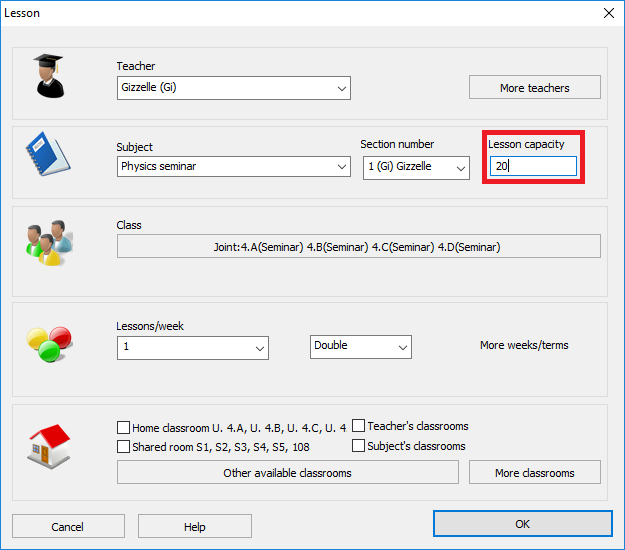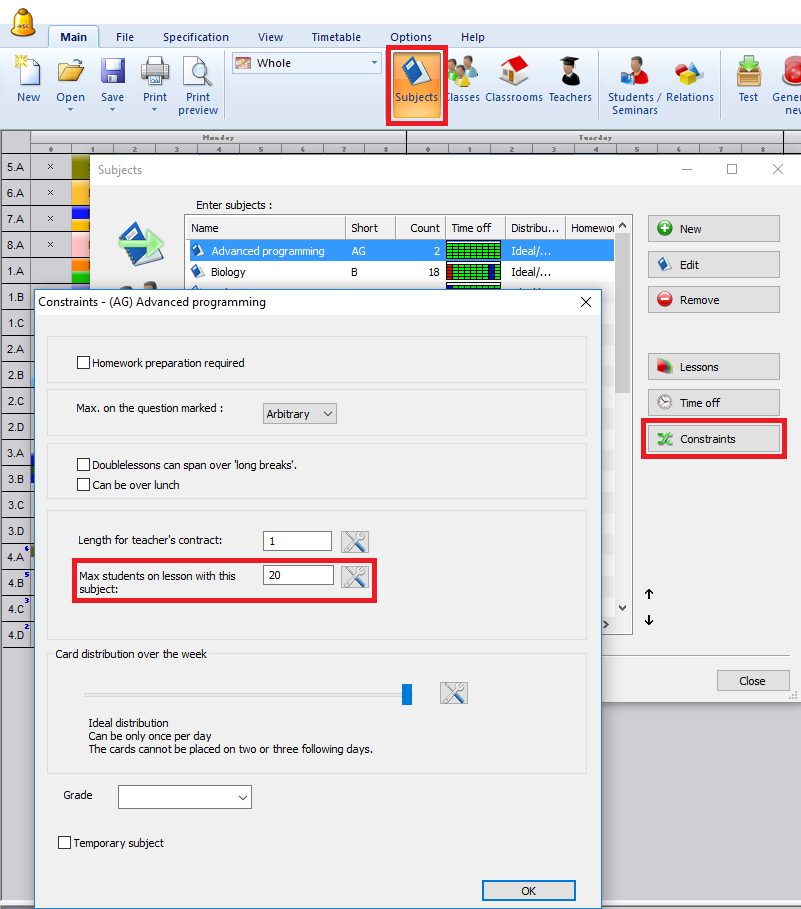#### aSc الجداول المدرسية - صفحة المساعدةaSc EduPage - Online HelpaSc Video HelpaSc الجداول المدرسية - Seminars/Courses

Max students for certain seminar lesson
هذا المقال متوفر حسب اللغات التالية :

It is possible to input max number of students that can be assigned to given seminar (section). You can input it in "Lesson" dialog in "Lesson capacity" field:Note: you can input both minimum and maximum capacity: if you input 10-20 this means that all solution where there are between 10 and 20 students are considered correct for the generator.
If you input only 20 - then this number indicates maximum. The minimum is calculated as number of students / number of sections +- 10%. This 10% can be specified in the seminar/settings section.

It is also possible to input this for all lessons of some subject in subject constraints:Note: Value for subject is used only for lessons where "Lesson capacity" field is left blank. If you input "Lesson capacity" in lesson, then value from subject is ignored for that lesson.تمت مشاهدة هذا المقال 51508 مرة40761 / 51508المقال السابق الرجوع الى صفحة المساعدة لدى aSc المقال التالي